# NCERT solutions for Mathematics Exemplar Class 6 chapter 9 - Symmetry and Practical Geometry [Latest edition]

#### Chapters## Chapter 9: Symmetry and Practical Geometry

Exercise
Exercise [Pages 136 - 145]

### NCERT solutions for Mathematics Exemplar Class 6 Chapter 9 Symmetry and Practical Geometry Exercise [Pages 136 - 145]

#### Choose the correct alternative:

Exercise | Q 1 | Page 136

In the following figures, the figure that is not symmetric with respect to any line is ______.

•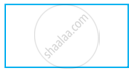•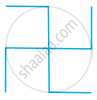•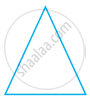•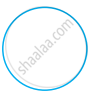Exercise | Q 2 | Page 136

The number of lines of symmetry in a scalene triangle is ______.

• 0

• 1

• 2

• 3

Exercise | Q 3 | Page 137

The number of lines of symmetry in a circle is ______.

• 0

• 2

• 4

• More than 4

Exercise | Q 4 | Page 137

Which of the following letters does not have the vertical line of symmetry?

• M

• H

• E

• V

Exercise | Q 5 | Page 137

Which of the following letters have both horizontal and vertical lines of symmetry?

• X

• E

• M

• K

Exercise | Q 6 | Page 137

Which of the following letters does not have any line of symmetry?

• M

• S

• K

• H

Exercise | Q 7 | Page 137

Which of the following letters has only one line of symmetry?

• H

• X

• Z

• T

Exercise | Q 8 | Page 137

The instrument to measure an angle is a ______.

• Ruler

• Protractor

• Divider

• Compasses

Exercise | Q 9 | Page 137

The instrument to draw a circle is ______.

• Ruler

• Protractor

• Divider

• Compasses

Exercise | Q 10 | Page 137

Number of set squares in the geometry box is ______.

• 0

• 1

• 2

• 3

Exercise | Q 11 | Page 137

The number of lines of symmetry in a ruler is ______.

• 0

• 1

• 2

• 4

Exercise | Q 12 | Page 137

The number of lines of symmetry in a divider is ______.

• 0

• 1

• 2

• 3

Exercise | Q 13 | Page 137

The number of lines of symmetry in compasses is ______.

• 0

• 1

• 2

• 3

Exercise | Q 14 | Page 137

The number of lines of symmetry in a protractor is ______.

• 0

• 1

• 2

• More than

Exercise | Q 15 | Page 138

The number of lines of symmetry in a 45° – 45° – 90° set-square is ______.

• 0

• 1

• 2

• 3

Exercise | Q 16 | Page 138

The number of lines of symmetry in a 30° – 60° – 90° set square is ______.

• 0

• 1

• 2

• 3

Exercise | Q 17 | Page 138

The instrument in the geometry box having the shape of a triangle is called a ______.

• Protractor

• Compasses

• Divider

• Set-square

#### Fill in the blank:

Exercise | Q 18 | Page 138

The distance of the image of a point (or an object) from the line of symmetry (mirror) is ______ as that of the point (object) from the line (mirror).

Exercise | Q 19 | Page 138

The number of lines of symmetry in a picture of Taj Mahal is ______.

Exercise | Q 20 | Page 138

The number of lines of symmetry in a rectangle and a rhombus are ______ (equal/unequal).

Exercise | Q 21 | Page 138

The number of lines of symmetry in a rectangle and a square are ______ (equal/unequal).

Exercise | Q 22 | Page 138

If a line segment of length 5 cm is reflected in a line of symmetry (mirror), then its reflection (image) is a ______ of length _______.

Exercise | Q 23 | Page 138

If an angle of measure 80° is reflected in a line of symmetry, then the reflection is an ______ of measure ______.

Exercise | Q 24 | Page 138

The image of a point lying on a line l with respect to the line of symmetry l lies on ______.

Exercise | Q 25 | Page 138

In figure, if B is the image of the point A with respect to the line l and P is any point lying on l, then the lengths of the line segments PA and PB are ______.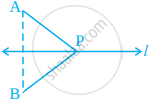Exercise | Q 26 | Page 139

The number of lines of symmetry in given figure is ______.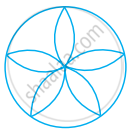Exercise | Q 27 | Page 139

The common properties in the two set-squares of a geometry box are that they have a ______ angle and they are of the shape of a ______.

Exercise | Q 28 | Page 139

The digits having only two lines of symmetry are ______ and ______.

Exercise | Q 29 | Page 139

The digit having only one line of symmetry is ______.

Exercise | Q 30 | Page 139

The number of digits having no line of symmetry is ______.

Exercise | Q 31 | Page 139

The number of capital letters of the English alphabets having only vertical line of symmetry is ______.

Exercise | Q 32 | Page 139

The number of capital letters of the English alphabets having only horizontal line of symmetry is ______.

Exercise | Q 33 | Page 139

The number of capital letters of the English alphabets having both horizontal and vertical lines of symmetry is ______.

Exercise | Q 34 | Page 139

The number of capital letters of the English alphabets having no line of symmetry is ______.

Exercise | Q 35 | Page 139

The line of symmetry of a line segment is the ______ bisector of the line segment.

Exercise | Q 36 | Page 139

The number of lines of symmetry in a regular hexagon is ______.

Exercise | Q 37 | Page 139

The number of lines of symmetry in a regular polygon of n sides is ______.

Exercise | Q 38 | Page 139

A protractor has ______ line/lines of symmetry.

Exercise | Q 39 | Page 140

A 30° – 60° – 90° set-square has ______ line/lines of symmetry.

Exercise | Q 40 | Page 140

A 45° – 45° – 90° set-square has ______ line/lines of symmetry.

Exercise | Q 41 | Page 140

A rhombus is symmetrical about ______.

Exercise | Q 42 | Page 140

A rectangle is symmetrical about the lines joining the ______ of the opposite sides.

#### State whether the following statement is True or False:

Exercise | Q 43 | Page 140

A right triangle can have at most one line of symmetry.

• True

• False

Exercise | Q 44 | Page 140

A kite has two lines of symmetry.

• True

• False

Exercise | Q 45 | Page 140

A parallelogram has no line of symmetry.

• True

• False

Exercise | Q 46 | Page 140

If an isosceles triangle has more than one line of symmetry, then it need not be an equilateral triangle.

• True

• False

Exercise | Q 47 | Page 140

If a rectangle has more than two lines of symmetry, then it must be a square.

• True

• False

Exercise | Q 48 | Page 140

With ruler and compasses, we can bisect any given line segment.

• True

• False

Exercise | Q 49 | Page 140

Only one perpendicular bisector can be drawn to a given line segment.

• True

• False

Exercise | Q 50 | Page 140

Two perpendiculars can be drawn to a given line from a point not lying on it.

• True

• False

Exercise | Q 51 | Page 140

With a given centre and a given radius, only one circle can be drawn.

• True

• False

Exercise | Q 52 | Page 140

Using only the two set-squares of the geometry box, an angle of 40° can be drawn.

• True

• False

Exercise | Q 53 | Page 140

Using only the two set-squares of the geometry box, an angle of 15° can be drawn.

• True

• False

Exercise | Q 54 | Page 140

If an isosceles triangle has more than one line of symmetry, then it must be an equilateral triangle.

• True

• False

Exercise | Q 55 | Page 140

A square and a rectangle have the same number of lines of symmetry.

• True

• False

Exercise | Q 56 | Page 140

A circle has only 16 lines of symmetry.

• True

• False

Exercise | Q 57 | Page 140

A 45° – 45° – 90° set-square and a protractor have the same number of lines of symmetry.

• True

• False

Exercise | Q 58 | Page 141

It is possible to draw two bisectors of a given angle.

• True

• False

Exercise | Q 59 | Page 141

A regular octagon has 10 lines of symmetry.

• True

• False

Exercise | Q 60 | Page 141

Infinitely many perpendiculars can be drawn to a given ray.

• True

• False

Exercise | Q 61 | Page 141

Infinitely many perpendicular bisectors can be drawn to a given ray.

• True

• False

Exercise | Q 62 | Page 141

Is there any line of symmetry in the figure? If yes, draw all the lines of symmetry.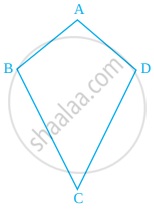Exercise | Q 63 | Page 141

In figure, PQRS is a rectangle. State the lines of symmetry of the rectangle.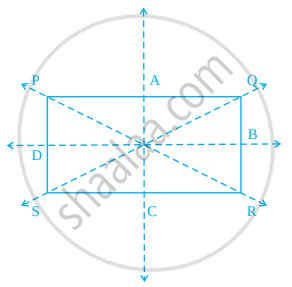Exercise | Q 64 | Page 142

Write all the capital letters of the English alphabets which have more than one lines of symmetry.

Exercise | Q 65 | Page 142

Write the letters of the word ‘MATHEMATICS’ which have no line of symmetry.

Exercise | Q 66 | Page 142

Write the number of lines of symmetry in each letter of the word ‘SYMMETRY’.

#### Match the following

Exercise | Q 67 | Page 142
 Shape Number of lines of symmetry (i) Isosceles triangle (a) 6 (ii) Square (b) 5 (iii) Kite (c) 4 (iv) Equilateral triangle (d) 3 (v) Rectangle (e) 2 (vi) Regular hexagon (f) 1 (vii) Scalene triangle (g) 0
Exercise | Q 68 | Page 142

Open your geometry box. There are some drawing tools. Observe them and complete the following table:

 Name of the tool Number of lines of symmetry (i) The Ruler ______ (ii) The Divider ______ (iii) The Compasses ______ (iv) The Protactor ______ (v) Triangular piece with two equal sides ______ (vi) Triangular piece with unequal sides ______
Exercise | Q 69 | Page 143

Draw the images of points A and B in line l of figure and name them as A’ and B’, respectively. Measure AB and A’B’. Are they equal?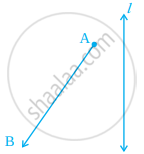Exercise | Q 70 | Page 143

In figure, the point C is the image of point A in line l and line segment BC intersects the line l at P.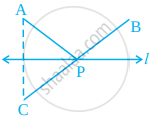(a) Is the image of P in line l the point P itself?
(b) Is PA = PC?
(c) Is PA + PB = PC + PB?
(d) Is P that point on line l from which the sum of the distances of points A and B is minimum?

Exercise | Q 71 | Page 143

Complete the figure so that line l becomes the line of symmetry of the whole figure.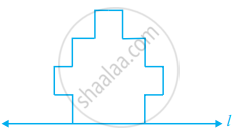Exercise | Q 72 | Page 143

Draw the images of the points A, B and C in the line m (figure). Name them as A’,B’ and C respectively and join them in pair. Measure AB, BC, CA, A’B’, B’C’ and C’A’. Is AB = A’B’, BC = B’C’ and CA = C’A’?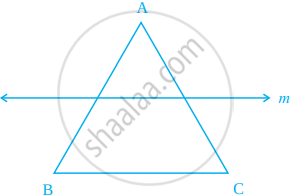Exercise | Q 73 | Page 144

Draw the images P’, Q’ and R’ of the points P, Q and R respectively in the line n. Join P’Q’ and Q’ R’ to form an angle P’Q’R’. Measure ∠PQR and ∠P’Q’R’. Are the two angles equal?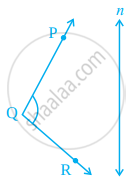Exercise | Q 74 | Page 144

Complete the figure by taking l as the line of symmetry of the whole figure.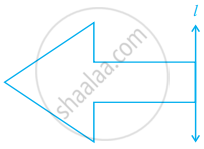Exercise | Q 75 | Page 144

Draw a line segment of length 7 cm. Draw its perpendicular bisector, using ruler and compasses.

Exercise | Q 76 | Page 144

Draw a line segment of length 6.5 cm and divide it into four equal parts, using ruler and compasses.

Exercise | Q 77 | Page 144

Draw an angle of 140° with the help of a protractor and bisect it using ruler and compasses.

Exercise | Q 78 | Page 144

Draw an angle of 65° and draw an angle equal to this angle, using ruler and compasses.

Exercise | Q 79 | Page 144

Draw an angle of 80° using a protractor and divide it into four equal parts, using ruler and compasses.Check your construction by measurement.

Exercise | Q 80 | Page 144

Copy figure on your notebook and draw a perpendicular to l through P, using (i) set squares (ii) protractor (iii) ruler and compass. How many such perpendiculars are you able to draw?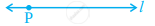Exercise | Q 81 | Page 145

Copy figure on your notebook and draw a perpendicular from P to line m using (i) set squares (ii) protractor (iii) ruler and compass. How many such perpendicular are you able to draw?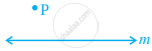Exercise | Q 82 | Page 145

Draw a circle of radius 6 cm using ruler and compasses. Draw one of its diameters. Draw the perpendicular bisector of this diameter. Does this perpendicular bisector contain another diameter of the circle?

Exercise | Q 83 | Page 145

Bisect ∠XYZ of figure.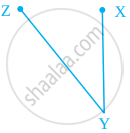Exercise | Q 84 | Page 145

Draw an angle of 60° using ruler and compasses and divide it into four equal parts. Measure each part.

Exercise | Q 85 | Page 145

Bisect a straight angle, using ruler and compasses. Measure each part.

Exercise | Q 86 | Page 145

Bisect a right angle, using ruler and compasses. Measure each part. Bisect each of these parts. What will be the measure of each of these parts?

Exercise | Q 87 | Page 145

Draw an angle ABC of measure 45°, using ruler and compasses. Now draw an angle DBA of measure 30o , using ruler and compasses as shown in figure. What is the measure of ∠DBC?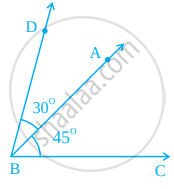Exercise | Q 88 | Page 145

Draw a line segment of length 6 cm. Construct its perpendicular bisector. Measure the two parts of theline segment.

Exercise | Q 89 | Page 145

Draw a line segment of length 10 cm. Divide it into four equal parts. Measure each of these parts.

## Chapter 9: Symmetry and Practical Geometry

Exercise## NCERT solutions for Mathematics Exemplar Class 6 chapter 9 - Symmetry and Practical Geometry

NCERT solutions for Mathematics Exemplar Class 6 chapter 9 (Symmetry and Practical Geometry) include all questions with solution and detail explanation. This will clear students doubts about any question and improve application skills while preparing for board exams. The detailed, step-by-step solutions will help you understand the concepts better and clear your confusions, if any. Shaalaa.com has the CBSE Mathematics Exemplar Class 6 solutions in a manner that help students grasp basic concepts better and faster.

Further, we at Shaalaa.com provide such solutions so that students can prepare for written exams. NCERT textbook solutions can be a core help for self-study and acts as a perfect self-help guidance for students.

Concepts covered in Mathematics Exemplar Class 6 chapter 9 Symmetry and Practical Geometry are Concept of Symmetry, Making Symmetric Figures: Ink-blot Devils, Concept of Lines Symmetry, Concept of Reflection Symmetry, Introduction to Practical Geometry, Construction of a Circle When Its Radius is Known, Construction of a Line Segment of a Given Length, Constructing a Copy of a Given Line Segment, Drawing a Perpendicular to a Line at a Point on the Line, Drawing a Perpendicular to a Line Through a Point Not on It, Drawing the Perpendicular Bisector of a Line Segment, Constructing an Angle of a Given Measure, Constructing a Copy of an Angle of Unknown Measure, Constructing a Bisector of an Angle, Angles of Special Measures - 30°, 45°, 60°, 90°, and 120°.

Using NCERT Class 6 solutions Symmetry and Practical Geometry exercise by students are an easy way to prepare for the exams, as they involve solutions arranged chapter-wise also page wise. The questions involved in NCERT Solutions are important questions that can be asked in the final exam. Maximum students of CBSE Class 6 prefer NCERT Textbook Solutions to score more in exam.

Get the free view of chapter 9 Symmetry and Practical Geometry Class 6 extra questions for Mathematics Exemplar Class 6 and can use Shaalaa.com to keep it handy for your exam preparation